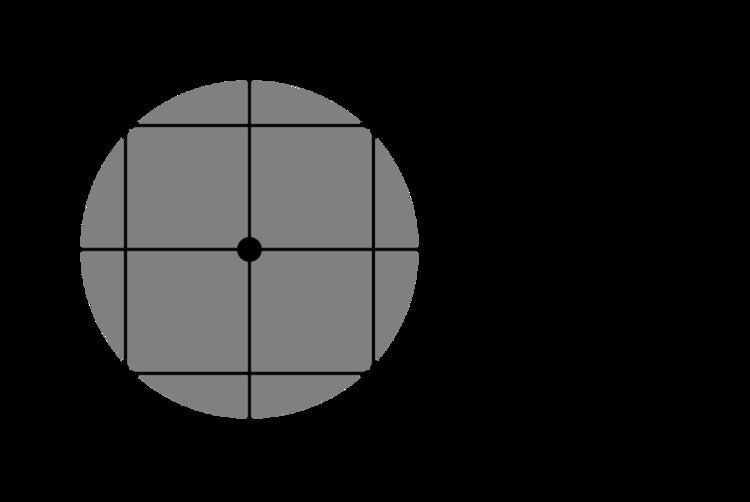# Point particle

Updated on
Covid-19A point particle (ideal particle or point-like particle, often spelled pointlike particle) is an idealization of particles heavily used in physics. Its defining feature is that it lacks spatial extension: being zero-dimensional, it does not take up space. A point particle is an appropriate representation of any object whose size, shape, and structure is irrelevant in a given context. For example, from far enough away, an object of any shape will look and behave as a point-like object.

## Contents

In the theory of gravity, physicists often discuss a point mass, meaning a point particle with a nonzero mass and no other properties or structure. Likewise, in electromagnetism, physicists discuss a point charge, a point particle with a nonzero charge.

Sometimes, due to specific combinations of properties, extended objects behave as point-like even in their immediate vicinity. For example, spherical objects interacting in 3-dimensional space whose interactions are described by the inverse square law behave in such a way as if all their matter were concentrated in their centers of mass. In Newtonian gravitation and classical electromagnetism, for example, the respective fields outside of a spherical object are identical to those of a point particle of equal charge/mass located at the center of the sphere.

In quantum mechanics, the concept of a point particle is complicated by the Heisenberg uncertainty principle, because even an elementary particle, with no internal structure, occupies a nonzero volume. For example, the atomic orbit of an electron in the hydrogen atom occupies a volume of ~10−30 m3. There is nevertheless a distinction between elementary particles such as electrons or quarks, which have no known internal structure, versus composite particles such as protons, which do have internal structure: A proton is made of three quarks. Elementary particles are sometimes called "point particles", but this is in a different sense than discussed above.

## Property concentrated at a single point

When a point particle has an additive property, such as mass or charge, concentrated at a single point in space, this can be represented by a Dirac delta function.

## Physical point mass

Point mass (pointlike mass) is the concept, for example in classical physics, of a physical object (typically matter) that has nonzero mass, and yet explicitly and specifically is--or is being thought of or modeled as--infinitesimal (infinitely small) in its volume or linear dimensions.

## Application

A common use for point mass lies in the analysis of the gravitational fields. When analyzing the gravitational forces in a system, it becomes impossible to account for every unit of mass individually. However, a spherically symmetric body affects external objects gravitationally as if all of its mass were concentrated at its center.

## Probability point mass

A point mass in probability and statistics does not refer to mass in the sense of physics, but rather refers to a finite nonzero probability that is concentrated at a point in the probability mass distribution, where there is a discontinuous segment in a probability density function. To calculate such a point mass, an integration is carried out over the entire range of the random variable, on the probability density of the continuous part. After equating this integral to 1, the point mass can be found by further calculation.

## Point charge

A point charge is an idealized model of a particle which has an electric charge. A point charge is an electric charge at a mathematical point with no dimensions.

The fundamental equation of electrostatics is Coulomb's law, which describes the electric force between two point charges. The electric field associated with a classical point charge increases to infinity as the distance from the point charge decreases towards zero making energy (thus mass) of point charge infinite.

Earnshaw's theorem states that a collection of point charges cannot be maintained in an equilibrium configuration solely by the electrostatic interaction of the charges.

## In quantum mechanics

In quantum mechanics, there is a distinction between an elementary particle (also called "point particle") and a composite particle. An elementary particle, such as an electron, quark, or photon, is a particle with no internal structure, whereas a composite particle, such as a proton or neutron, has an internal structure (see figure). However, neither elementary nor composite particles are spatially localized, because of the Heisenberg uncertainty principle. The particle wavepacket always occupies a nonzero volume. For example, see atomic orbital: The electron is an elementary particle, but its quantum states form three-dimensional patterns.

Nevertheless, there is good reason that an elementary particle is often called a point particle. Even if an elementary particle has a delocalized wavepacket, the wavepacket is in fact a quantum superposition of quantum states wherein the particle is exactly localized. This is not true for a composite particle, which can never be represented as a superposition of exactly-localized quantum states. It is in this sense that physicists can discuss the intrinsic "size" of a particle: The size of its internal structure, not the size of its wavepacket. The "size" of an elementary particle, in this sense, is exactly zero.

For example, for the electron, experimental evidence shows that the size of an electron is less than 10−18 m. This is consistent with the expected value of exactly zero. (This should not be confused with the classical electron radius, which, despite the name, is unrelated to the actual size of an electron.)

Topics